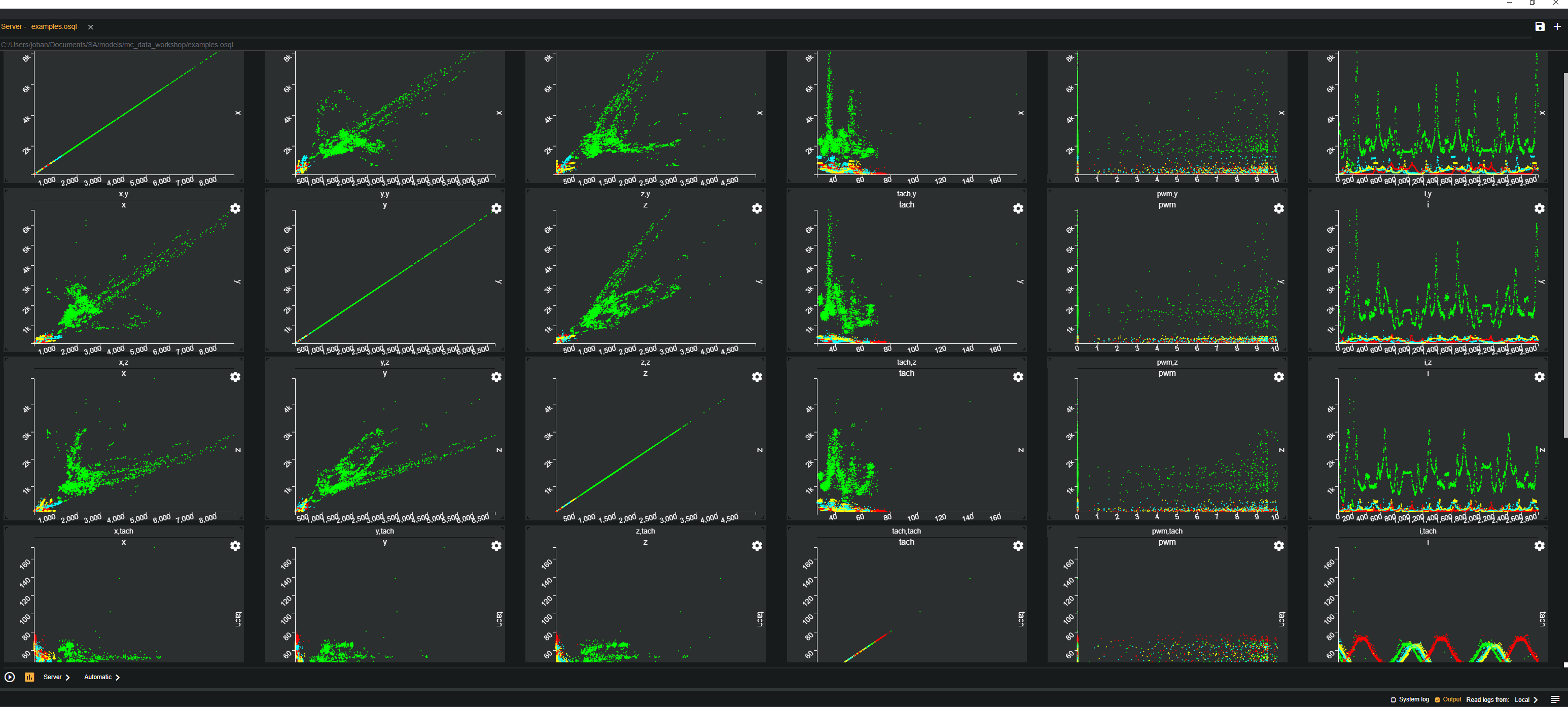# Statistical analysis of large datasetsDo you want to learn how to create awesome looking charts and get a statistical understanding of your dataset using plots like the one above? Then this guide is for you!

This tutorial will walk you through how to do statistical analysis of a dataset. This dataset is a collection of data from a Computer Fan where each reading has five measurements (observable variables): Accelerometer (X, Y, Z), Tachometer, and PWM.

This is a walk through of the work flow from a raw dataset to understanding the data. The first two parts will walk through the first steps of converting the raw data to a streamed format and looking at it. Below is an overview of the steps:

Note

If you are running in SA Studio Community Edition or do not want to wait for the processing of all histograms you can go straight to step 5 since this model comes with the statistics pre-loaded.

1. Creating a meta data model for the dataset
2. Converting JSON-object dataset into a stream of Vectors.
3. Looking at the raw dataset - can we see some obvious patterns?
4. Calculating statistics over the dataset for further analysis.
• Global stats (mean, standard deviation, min, max, count) for each measurement.
• Histograms of all variables and classes.
• Windowed statistics to compare variables.
5. Making sense of the data.
• Using Parallel coordinates and Scatter Plots
• Using Scatter plot Matrices
• Comparing histograms
6. Final words.

## The dataset​

The dataset can be downloaded here. It has already been transformed into a streaming format as section 1 in Pre processing describes.

• Accelerometer - a regular accelerometer with x, y, and z measurements.
• Tachometer measures how often the fan does a full Revolution.
• PWM - Pulse width modifier, control signal to the motor.

### Classes:​

• Low voltage - low voltage supply to the fan (10V istead of 12V).
• Normal.
• Object - small electrical cord stuck in fan.
• Obstructed - (cloth put in front of fan to simulate dust build up.